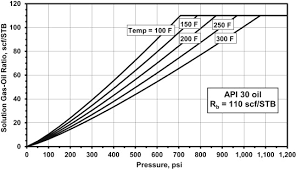## How to Calculate and Solve for Vasquez-Beggs Gas Solubility in a Fluid | The Calculator EncyclopediaThe image above represents the Vasquez-Beggs Gas Solubility.

To compute for the Vasquez-Beggs gas solubility, five essential parameters are needed and these parameters are API Gravity (°API), Temperature (°Rankine) (T), Gas Gravity at Reference Separator Pressure (γgs), Gas Gravity at Actual Separator Psep and Tsep g) and Gas Solubility Parameter (C1, C2, C3).

The formula for calculating Vasquez-Beggs gas solubility:

Rs = C1 γgs γgC2 exp[C3(°API / T)]

Where:

Rs = Vasquez-Beggs Gas Solubitity
°API = API Gravity
T = Temperature (°Rankine)
γgs = Gas Gravity at Reference Separator Pressure
γg = Gas Gravity at Actual Separator Psep and Tsep
C1, C2, C3 = Gas Solubility Parameter

Let’s solve an example;
Find the Vasquez-Beggs gas solubility when the API Gravity is 24, Temperature is 180, gas gravity at reference separator pressure is 60, gas gravity at actual separator is 56, gas solubility parameter is 72, 66 and 45.

This implies that;

°API = API Gravity = 24
T = Temperature (°Rankine) = 180
γgs = Gas Gravity at Reference Separator Pressure = 60
γg = Gas Gravity at Actual Separator Psep and Tsep = 56
C1, C2, C3 = Gas Solubility Parameter = 72, 66, 45

Rs = C1 γgs γgC2 exp[C3(°API/ T)]
Rs = 72 x 60 x 5666 exp[45(24/ 180)]
Rs = 72 x 60 x 5666 exp[45(0.133)]
Rs = 72 x 60 x 5666 exp
Rs = 72 x 60 x 2.401e+115 exp
Rs = 72 x 60 x 2.401e+115 x 403.428
Rs = 6.974e+119

Therefore, the Vasquez-Beggs gas solubility is 6.974e+119.